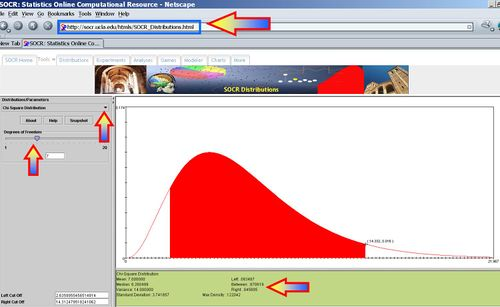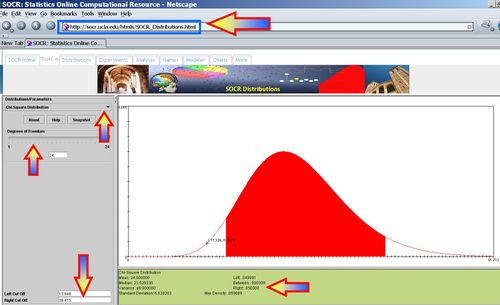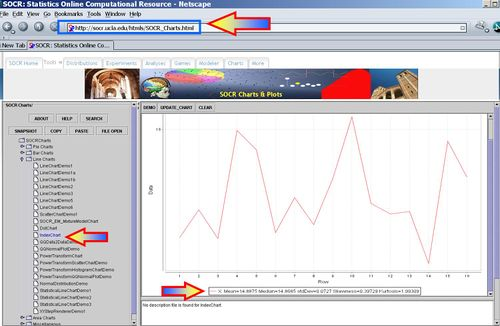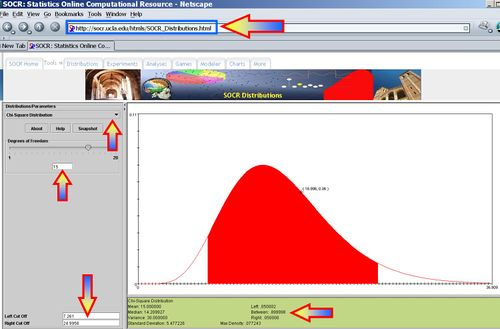Difference between revisions of "AP Statistics Curriculum 2007 Estim Var"

General Advance-Placement (AP) Statistics Curriculum - Estimating Population Variance

In manufacturing, and many other fields, controlling the amount of variance in producing machinery parts is very important. It is important that the parts vary little or not at all.

Point Estimates of Population Variance and Standard Deviation

The most unbiased point estimate for the population variance $$\sigma^2$$ is the sample-variance (s2) and the point estimate for the population standard deviation $$\sigma$$ is the sample standard deviation (s).

We use a Chi-square distribution to construct confidence intervals for the variance and standard distribution. If the process or phenomenon we study generates a Normal random variable, then computing the following random variable (for a sample of size $$n>1$$) has a Chi-square distribution $\chi_o^2 = {(n-1)s^2 \over \sigma^2}$

Chi-square Distribution Properties

• All chi-squares values $$\chi_o^2 \geq 0$$.
• The chi-square distribution is a family of curves, each determined by the degrees of freedom (n-1). See the interactive Chi-Square distribution.
• To form a confidence interval for the variance ($$\sigma^2$$), use the $$\chi^2(df=n-1)$$ distribution with degrees of freedom equal to one less than the sample size.
• The area under each curve of the Chi-square distribution equals one.
• All Chi-square distributions are positively skewed.Interval Estimates of population Variance and Standard Deviation

Notice that the Chi-square distribtuion is not symmetric (positively skewed) and therefore, there are two critical values for each level of confidence. The value $$\chi_L^2$$ represents the left-tail critical value and $$\chi_R^2$$ represents the rightt-tail critical value. For various degrees of freedom and areas, you can compute all critical values either using the SOCR Distributions or using the SOCR Chi-square distribution calculator.

• Example: Find the critical values, $$\chi_L^2$$ and $$\chi_R^2$$, for a 95% confidence interval when the sample size is 25. Use the following Protocol:
• Identify the degrees of freedom ($$df=n-1=24$$) and the level of confidence ($${\alpha\over 2}=0.025$$).
• Find the left and right critical values, $$\chi_L^2=13.848$$ and $$\chi_R^2=36.415$$, as in the image below.Confidence interval for $$\sigma^2$$

${(n-1)s^2 \over \chi_R^2} \leq \sigma^2 \leq {(n-1)s^2 \over \chi_L^2}$

Confidence interval for $$\sigma$$

$\sqrt{(n-1)s^2 \over \chi_R^2} \leq \sigma \leq \sqrt{(n-1)s^2 \over \chi_L^2}$

Hands-on activities

• Construct the confidence intervals for $$\sigma^2$$ and $$\sigma$$ assuming the observations below represent a random sample from the liquid content (in fluid ounces) of 16 beverage cans and can be considered as Normally distributed. Use a 90% level of confidence.
 14.816 14.863 14.814 14.998 14.965 14.824 14.884 14.838 14.916 15.021 14.874 14.856 14.86 14.772 14.98 14.919
• Get the sample statistics from SOCR Charts (e.g., Index Plot); Sample-Mean=14.8875; Sample-SD=0.072700298, Sample-Var=0.005285333.• Identify the degrees of freedom ($$df=n-1=15$$) and the level of confidence ($${\alpha \over 2}=0.05$$, as we are looking for a $$(1-\alpha)100% CI(\sigma^2)$$).
• Find the left and right critical values, $$\chi_L^2=7.261$$ and $$\chi_R^2=24.9958$$, as in the image below.